# BLAZE Multiphysics™ Models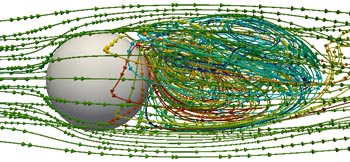Pressure Based Coupled Navier-Stokes Model - A pressure based Navier-Stokes solver which solves the Navier-Stokes equations or a subset thereof in a coupled fashion (all equations are solved simultaneously using a single sparse linear system) in order to maximize computational efficiency and convergence rate.Poisson Equation Based Wall Distance Model - A model which can be used to calculate the minimum distance to viscous walls in complex, arbitrary geometries in order to close certain turbulent kinetic energy transport equation formulations.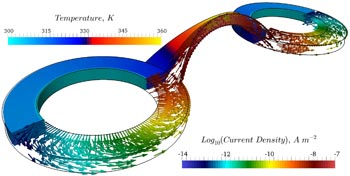Resistive Heating Model - A model which can be used to simulate current density and the associated Joule (resistive) heating in various materials. The materials property model can be used to specify resistivity, thermal conductivity, and other material properties as a function of material temperature or other variables. Conductors simulated using the Joule heating model may be thermally coupled to fluid simulations using the Grid Split Diffusion Model.

</div><div class='component-row'>Generalized Diffusion Model - A model which can be used to simulate generalized diffusion in any medium. In order to apply the model to a specific scenario, users may specify the diffusion equation internal dependent variables, any number of internal transient term coefficient variables, the diffusion coefficient internal variable, and both constant and linear source terms variables for maximum stability.Grid Split Diffusion Model - A model which can be used to simulate diffusion across grid splits. Grid splits can be used to separate an input grid into various sub-domains such that certain user specified models are solved on the entire domain while other user specified models are solved on the user-defined sub-domains. Models may be instantiated multiple times within a simulation. For example, users may use two Navier-Stokes models to simulate a reactive, compressible gas, and an incompressible liquid separated by a finite-thickness solid with user specified temperature dependent properties. In this scenario, the Grid Split Diffusion Model may be used to simulate thermal diffusion between the three user defined sub-domains.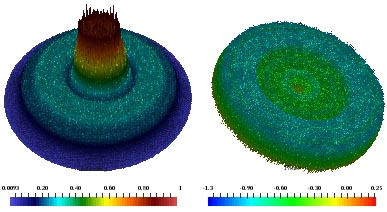Wave-Optics Model (Alpha) - A model which can be used to model the propagation of optical signals (including signal intensity and phase profiles) through dynamic media in two or three dimensions in a manner consistent with the wave equation. Various user specified curved optics and apertures may be included in simulations.

</div><div class='component-row'>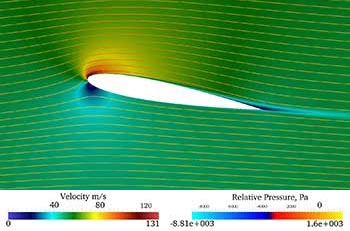One- and Two-Equation Turbulence Models - Various versatile one- and two- equation turbulence models which can be used to model turbulent dynamic viscosity, turbulent thermal diffusivity, or turbulent molecular diffusivity when coupled with over models.Molecular Transport Model - A model which can be used to model advection/diffusion based transport of neutral (uncharged) species, either advection/diffusion or drift/diffusion based transport of ion (charged) species and electrons, volume and surface gas- and plasma-kinetic (assuming electron energy equilibrium or non-equilibrium) reactions, volume photo-kinetic reactions when coupled to an optical model, and dynamically compute local fluid properties (e.g. viscosity, thermal conductivity, heat capacity, etc...) based on local specie mixtures.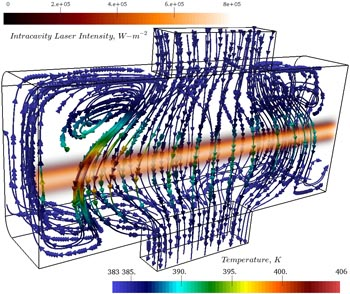Radiation Transport Model - A model which can be used to model the transport of photons in physical media along any user specified number of discrete propagation vectors or solid angle domains including interaction with any number of user specified boundary reflections and transmissions and interaction with non-equilibrium molecular dynamics via user specified photo-kinetic mechanisms (e.g. spontaneous emission, absorption, stimulated emission, etc...).

</div><div class='component-row'>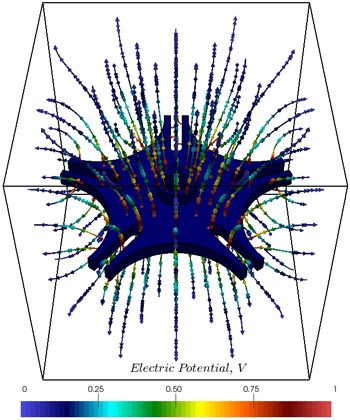Electric Field Model - A model which can be used to solve for the electric field in arbitrary geometries given boundary applied electric potentials, dielectric regions, and dynamic volume charges (when coupled to the molecular transport model).Material Properties Model - A model which can be used to apply user specified material property fits (e.g. constant, linear, polynomial, discrete data, functional equations, etc.) to any desired calculation property (e.g. viscosity, dielectric constant, heat-capacity, etc...) as a function of time, position, or other calculation properties (e.g. temperature, pressure, specie concentration, etc...).